# Schreiber Introduction to Higher Supergeometry

Contents

Abstract. Due to the existence of a) gauge fields and b) fermion fields, the geometry of physics is higher supergeometry, i.e. super-geometric homotopy theory. This is made precise via Grothendieck‘s functorial geometry implemented in higher topos theory. We give an introduction to the higher topos of higher superspaces and how it accomodates higher Lie theory of super L-∞ algebras. We close by indicating how geometric homotopy theory reveals that the superpoint emerges “from nothing”, and that core structure of M-theory emerges out of the superpoint via a sequence of invariant universal higher central extensions. This will be discussed in more detail in other talks in the meeting, notably in

• Hisham Sati, Geometric and topological aspects of M-branes

all summed up in the proceedings contribution:

# Contents

## Motivation

$\phantom{AA}$ physics $\phantom{AA}$$\phantom{AA}$ mathematics $\phantom{AA}$
$\phantom{AA}$ gauge principle $\phantom{AA}$$\phantom{AA}$ higher geometry $\phantom{AA}$
$\phantom{AA}$ (geometric homotopy theory) $\phantom{AA}$
$\phantom{AA}$ Pauli exclusion principle $\phantom{AA}$$\phantom{AA}$ supergeometry $\phantom{AA}$

Here:

1. Supergeometry is geometry whose spaces may have algebras of functions that are $\mathbb{Z}_2$-graded-commutative algebras. This is the mathematical reflection of the Pauli exclusion principle, which says that a fermionic wave function $\Psi$ on a phase space of a physical system with fermions has to have vanishing square. By linearity this implies that

\begin{aligned} 0 & = (\Psi_1 + \Psi_2)^2 \\ & = \underset{ = 0}{\underbrace{ (\Psi_1)^2 }} + \underset{ = 0}{\underbrace{ \Psi_1 \Psi_2 + \Psi_2 \Psi_1 }} + \underset{ = 0}{\underbrace{ (\Psi_2)^2 }} \end{aligned}

and hence that fermionic wave functions anti-commute, and hence are the odd-graded elements in a commutative superalgebra (a slightly noncommutative algebra!)

Ever since the existence of fermionic particles was experimentally established, around the time of the Stern-Gerlach experiment in the 1920s, it is thus an experimental fact that fundamental physics is described by supergeometry. (This is not necessarily super-symmetric, though of course there is a close relation.)

2. Higher structures is short for higher homotopy theoretic structures and reflects the gauge principle of physics: This says that, generally, it does not make invariant sense to ask if any two things $x$, $y$ (e.g. field histories) are equal, instead one must ask for a gauge transformation between them, mathematically a homotopy:

$x \stackrel{\gamma}{\longrightarrow} y$

This principle applies also to gauge transformations themselves, and thus leads to gauge-of-gauge transformations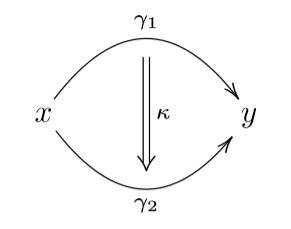and so on to ever higher gauge transformations: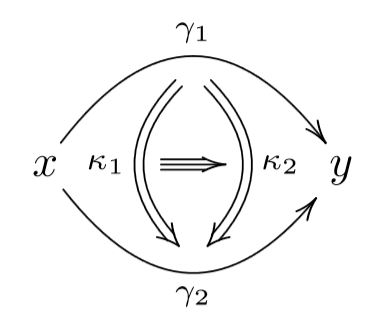mathematically reflected by higher homotopies in higher homotopy types.

Ever since the existence of gauge fields was understood in the 1920s, it is thus an experimental fact that fundamental physics is described by higher geometry.

A striking consequence is that, both in higher geometry as well as in supergeometry and therefore in the geometry of fundamental physics, spaces generally are not sets of points, as in the traditional definition of topological spaces or differentiable manifolds.

What, then, is the geometry of fundamental physics?

The right framework to answer questions like this has been urged by Alexander Grothendieck already in Grothendieck 73 (see Lawvere 03) and has been much expanded on by William Lawvere (e.g. Lawvere 97, Lawvere 91) and has an evident lift to higher geometry (Lurie 09, S. 13), but has remained somewhat of a “public secret”:

The answer is known, alternatively, as (higher) functorial geometry (Grothendieck) or synthetic differential geometry in gros toposes (Lawvere), or variants thereof.

In this lecture series we try to give a quick but self-contained introduction to higher differential supergeometry this way, following Schreiber 13.

We begin at the beginning, with introducing relevant basics of category theory, topos theory and homotopy theory. As a running example, we incrementally construct the higher topos of higher supergeometry. Along the way we provide pointers to applications in perturbative quantum field theory and the theory of fundamental super p-branes.

$\,$

$\,$

In view of M-theory we close by briefly highlighting the following phenomena, which are being expanded on in other talks at the meeting.

$\,$

$\;$ Super p-Brane Theory emerging from Super Homotopy Theory

$\;$ talk at String Math 2017, Hamburg (slides, expo, video)

$\,$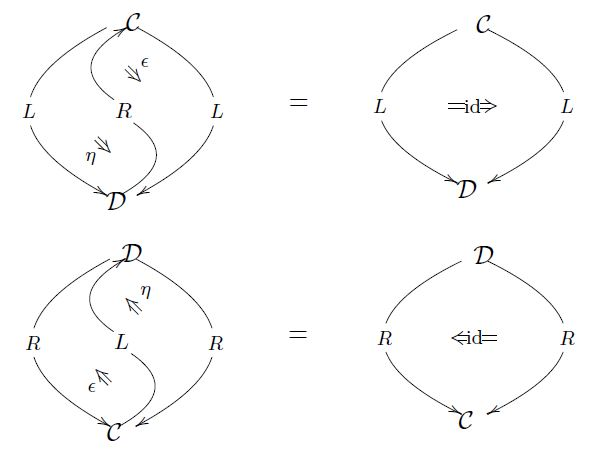1) category theory is really the theory of duality, formalized in the guise of adjunctions, hence adjoint pairs, and more generally adjoint triples for “dualities of dualities” (Lawvere 69, Lambek 82).

Moreover, this does capture duality in string theory.

Notably double dimensional reduction of fundamental super p-branes is given by the right base change adjunction along the point inclusion $\ast \to \mathbf{B}T$ into the moduli stack of torus-principal bundles. This fact gives rise to: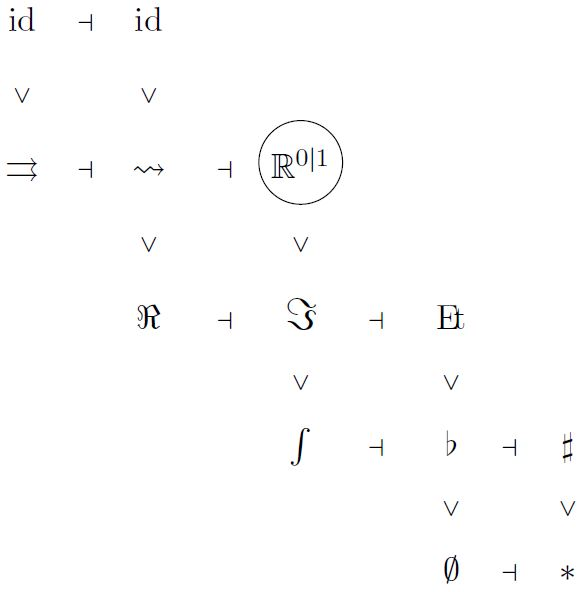2) supergeometry is stratified by a system of adjoint modalities ("super-differential cohesion") that progresses from the initial object $\emptyset$ to homotopy localization at the superpoint $\mathbb{R}^{0 \vert 1}$.

Such an abstract characterization of higher supergeometry provides a powerful means to reason about complicated phenomena such as torsion-free G-structures on supergeometric orbifolds by means of modal homotopy type theory (Wellen 18).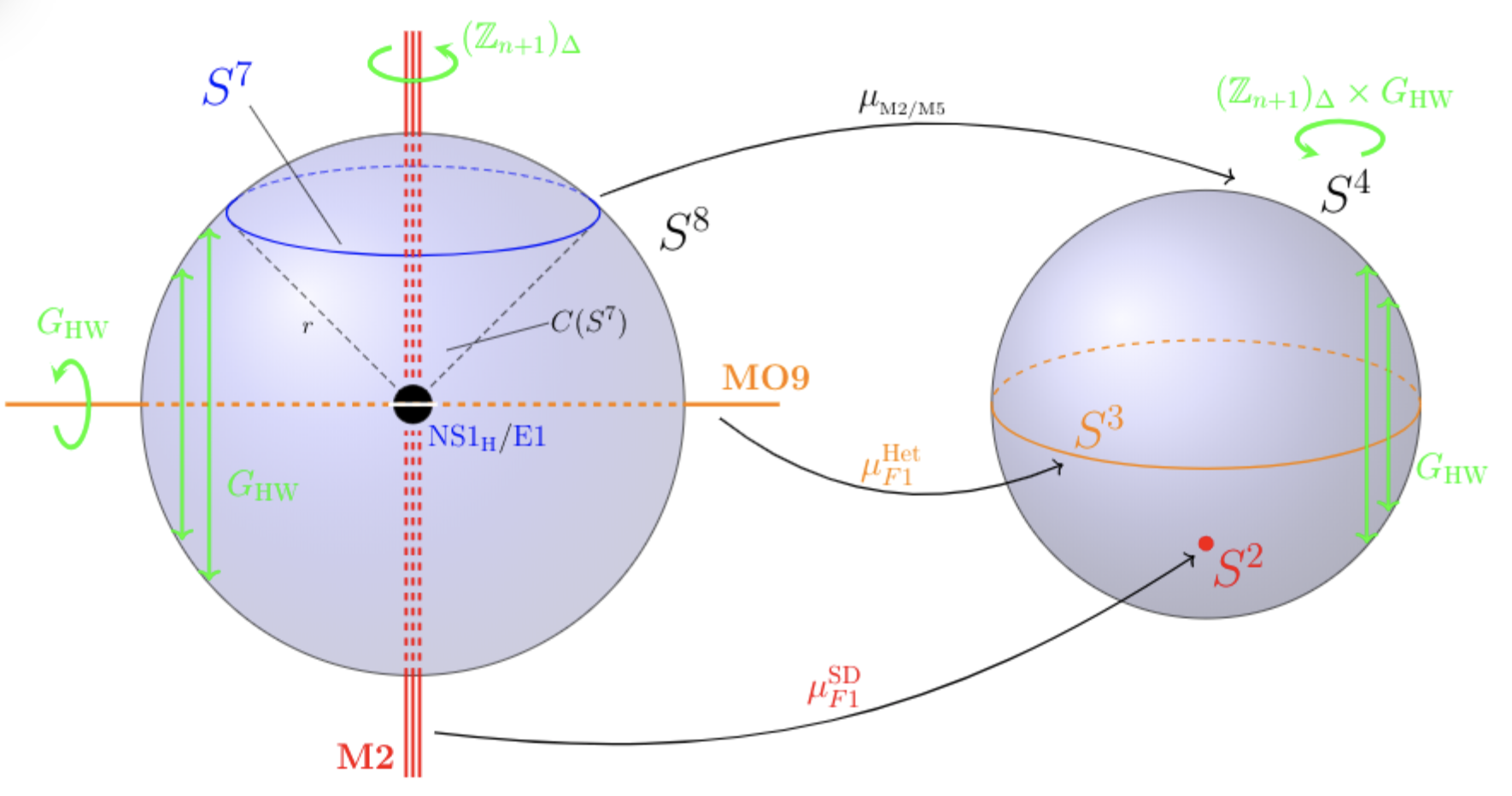This is necessary for studying global properties of black M-branes at ADE-singularities (Huerta-Sati-S. 18, see also the talk by H. Sati (here) at the meeting).

$\,$

$\,$

3) out of the superpoint emerges spacetime and the brane bouquet, by regarding the superpoint as a super L-∞ algebra and then applying the microscope of homotopy theory – the Postnikov-Whitehead tower (Fiorenza-Sati-S. 13, Huerta-S. 17, see the talks by J. Huerta (here) and H. Sati (here) at the meeting):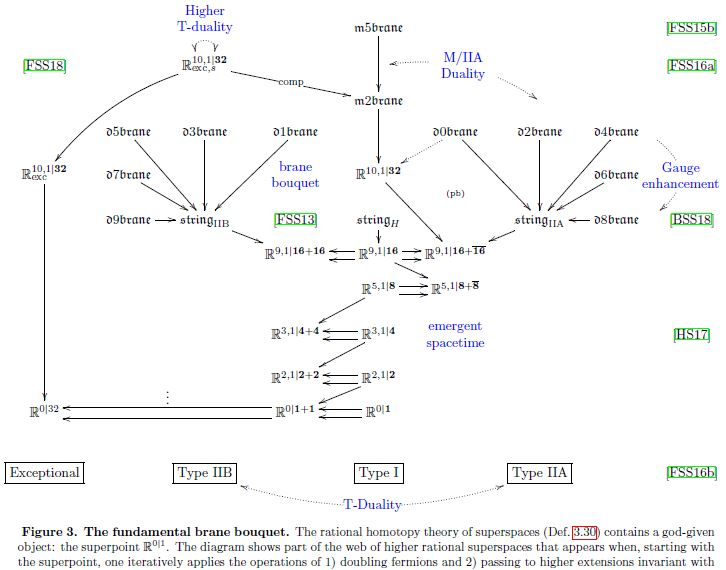(graphics taken from Huerta-Sati-S. 18)

$\,$

$\,$

## Lecture notes

Details behind the topics of the talk are in these two chapters from the lecture notes geometry of physics:

For further lecture notes on applications see these two chapters:

$\,$

$\,$

## Slides

then use ‘page down’ from here on

$\,$

$\,$

$\,$

$\,$

$\,$

$\,$

$\,$

$\,$

$\,$

$\,$

$\,$

$\array{ \text{adjust screen size from "3) Slides" to here} \\ \Downarrow}$

$\,$

Abstract. Due to the existence of a) gauge fields and b) fermion fields, the geometry of physics is higher supergeometry, i.e. super-geometric homotopy theory. This is made precise via Grothendieck‘s functorial geometry implemented in higher topos theory. We give an introduction to the higher topos of higher superspaces and how it accomodates higher Lie theory of super L-∞ algebras. We close by indicating how geometric homotopy theory reveals that the superpoint emerges “from nothing”, and that core structure of M-theory emerges out of the superpoint, as will be discussed in more detail in other talks in the meeting.

$\,$

$\,$

$\,$

$\,$

### 1) Duality theory

$\,$

$\,$

$\,$

We are concerned with duality.

$\phantom{AAA}$Informally:

$\phantom{AAA}$Two complementary perspectives whose unity reveals deeper reality.

$\,$

For instance:

1. in the theory of everything of 19th century philosophy:

duality of opposites in the Science of Logic;

2. in the theory of everything of 20th and 21st century physics:

$\,$

$\,$

$\,$

Public secret:

$\phantom{AAA}$Mathematical formalization of duality is

$\phantom{AAA}$ adjunction, adjoint equivalence, adjoint modality

$\phantom{AAA}$The theory of duality/adjunction is category theory.

$\phantom{AAA}$(Lambek 82, Lawvere 69).

$\phantom{AAA}$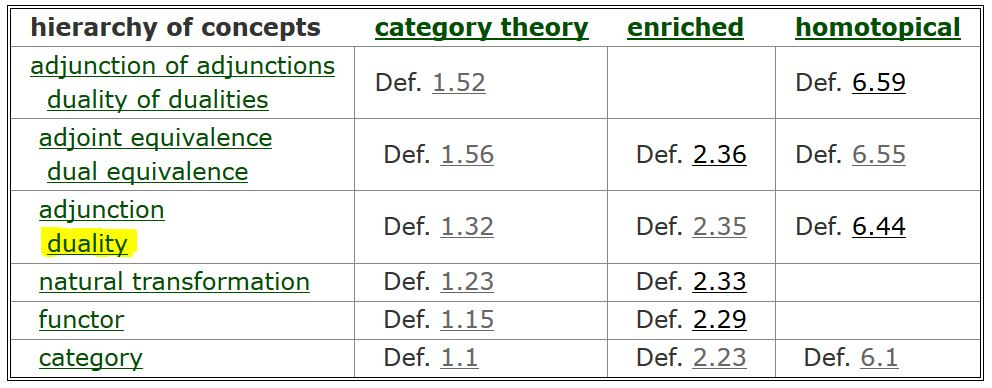$\,$

$\,$

$\,$

$\,$

A category of objects is

$\phantom{AAA}$a set (or class) of elements,

$\phantom{AAA}$but remembering how these may be mapped to each other,

$\phantom{AAA}$the (homo-)morphisms between them

$\phantom{AAA}$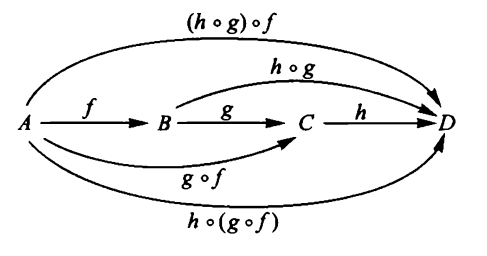structuralism:

$\phantom{AAA}$nature of objects in a category

$\phantom{AAA}$is reflected in their sets of morphisms to all other objects.

$\,$

$\,$

$\,$

$\,$

$\,$

$\,$

Examples of concrete categories

$\phantom{AAA}$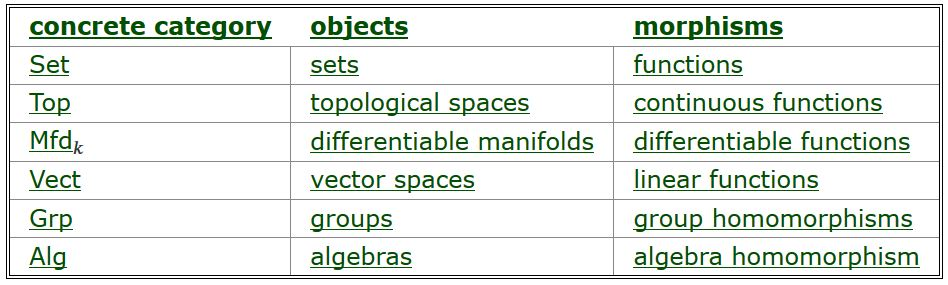$\,$

$\,$

$\,$

$\,$

$\,$

$\,$

$\,$

A functor between two categories

$\phantom{AAA}$is a function between their objects and morphisms

$\phantom{AAA}$which preserves the composition of morphisms.

$\,$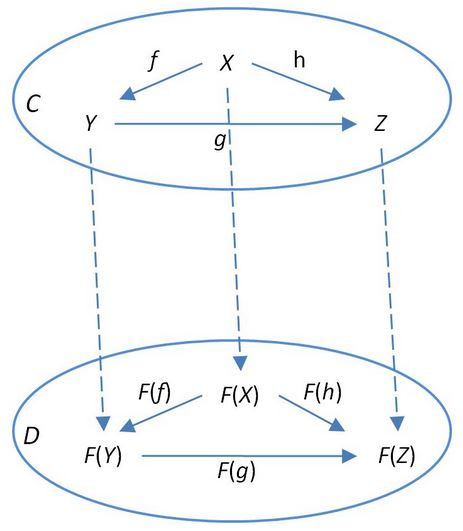$\,$

$\,$

$\,$

$\phantom{AAA}$$\array{ \mathcal{C} \\ {}^{\mathllap{F}}\Big\downarrow \\ \mathcal{D} }$

$\,$

$\,$

$\,$

$\,$

$\,$

Example. Adding a compact dimension is

$\phantom{AAA}$an endofunctor on the category of topological spaces

$\phantom{AAA}$$\array{ TopologicalSpaces & \overset{ \phantom{AA} S^1 \times (-) \phantom{AA} }{\longrightarrow} & TopologicalSpaces \\ \\ X &\mapsto& X \times S^1 \\ {}^{\mathllap{ f }}\Big\downarrow && \Big\downarrow{}^{ \mathrlap{ f \times \left( id_{S^1}\right) } } \\ Y &\mapsto& Y \times S^1 }$

$\,$

$\phantom{AAA}$This means that if

$\phantom{AAAAA}$$\Sigma_{p} \overset{\phantom{AA}\Phi\phantom{AA}}{\longrightarrow} X$

$\phantom{AAA}$is a p-brane worldvolume in $X$, then

$\phantom{AAAAA}$$\Sigma_{p} \times S^1 \overset{ \phantom{AA} \Phi \times \left(id_{S^1}\right) \phantom{AA} }{\longrightarrow} X \times S^1 \phantom{AAAAAAAAAAAAAAAAAAAAAAAAAAAAAAAAAAAAAAAAAAAAA}$

$\phantom{AAA}$is the corresponding (p+1)-brane worldvolume in $S^1 \times X$

$\phantom{AAA}$which wraps the compact fiber space.

$\,$

$\,$

ExampleForming free loop spaces is

$\phantom{AAA}$an endofunctor on the category of topological spaces

$\phantom{AAA}$$\array{ TopologicalSpaces & \overset{ \phantom{A} Maps(S^1,-) \phantom{A} }{\longrightarrow} & TopologicalSpaces \\ \\ X &\mapsto& Maps(S^1,X) \\ {}^{\mathllap{ f }}\Big\downarrow && \Big\downarrow{}^{ \mathrlap{ (-) \circ f } } \\ Y &\mapsto& Maps(S^1, Y) }$

$\,$

$\phantom{AAA}$This means that if

$\phantom{AAAAA}$$X \overset{\phantom{AA} f \phantom{AA}}{\longrightarrow} Y$

$\phantom{AAA}$is a map of target spaces

$\phantom{AAA}$for instance a KK-compactification-projection, then

$\phantom{AAAAA}$$Maps(S^1,X) \overset{\phantom{AA} Maps(S^1,f) \phantom{AA}}{\longrightarrow} Maps(S^1,Y)$

$\phantom{AAA}$is the corresponding map of closed string configuration spaces

$\,$

$\,$

$\,$

$\phantom{AAAAA}$ $\mathcal{C} \underoverset {\underset{\phantom{AAAA}G\phantom{AAAA}}{\longrightarrow}} {\overset{\phantom{AAAA}F\phantom{AAAA}}{\longrightarrow}} {\phantom{AAA}\phantom{\eta}\Downarrow \eta\phantom{AAA}} \mathcal{D}$

$\,$

$\phantom{AAA}$is an indexed set of morphisms between images of functors

$\phantom{AAAAA}$$\phantom{\array{X && }} F(X) \overset{\phantom{AA}\eta_X\phantom{AA}}{\longrightarrow} G(X)$

$\,$

$\phantom{AAA}$that respects composition on both sides.

$\phantom{AAAAA}$$\array{ X && F(X) &\overset{ \phantom{AA}\eta_X \phantom{AA} }{\longrightarrow}& G(X) \\ {}^{\mathllap{f}}\Big\downarrow &\phantom{AAA}& {}^{\mathllap{F(f)}}\Big\downarrow && \Big\downarrow{}^{\mathrlap{ G(f) }} \\ Y && F(Y) &\underset{ \phantom{AA}\eta_Y\phantom{AA} }{\longrightarrow}& G(Y) }$

$\,$

$\,$

$\,$

Example.Brane wrapping circle fiber

$\phantom{AAA}$Every topological space maps into

$\phantom{AAA}$the free loop space of its Cartesian product with the circle

$\phantom{AAAAA}$$\array{ X &\overset{ \phantom{AA}\eta_X\phantom{AA} }{\longrightarrow}& Maps\big( S^1, \; S^1 \times X \big) \\ x &\mapsto& (s \mapsto (s,x)) }$

$\phantom{AAA}$by sending each point to the loop which wraps the compact fiber space $S^1$ above it.

$\,$

$\phantom{AAA}$Conversely, there is the evaluation map

$\phantom{AAAAA}$$\array{ S^1 \times Maps(S^1, X) &\overset{ \epsilon_X }{\longrightarrow}& X \\ (s,\gamma) &\mapsto& \gamma(s) }$

$\,$

$\phantom{AAA}$These are natural transformations

$\phantom{AAAAA}$$TopologicalSpaces \underoverset {\underset{Maps(S^1,S^1 \times (-))}{\longrightarrow}} {\overset{\phantom{AAA}id\phantom{AAA}}{\longrightarrow}} {\phantom{AAAA}\Downarrow \eta\phantom{AAA}} TopologicalSpaces \underoverset {\underset{\phantom{AAA}id\phantom{AAA}}{\longrightarrow}} {\overset{ S^1 \times Maps(S^1, -) }{\longrightarrow}} {\phantom{AAAA}\Downarrow \epsilon\phantom{AAA}} TopologicalSpaces$

$\,$$\,$

$\phantom{AAA}$is a pair of functors back and forth

$\phantom{AAAA} \mathcal{D} \underoverset {\underset{R}{\longrightarrow}} {\overset{L}{\longleftarrow}} {\phantom{AA}\bot\phantom{AA}} \mathcal{C}$

$\phantom{AAA}$equipped with
$\phantom{AAA}$natural transformations

• $c \overset{\phantom{AA}\eta_c\phantom{AA}}{\longrightarrow} R L(c)$ (“unit”)

• $L R(d) \overset{ \phantom{AA}\epsilon_d\phantom{AA} }{\longrightarrow} d$ (“counit”)

$\phantom{AAA}$satisfying “zig-zag identity
$\phantom{AAA}$as shown on the right.

$\phantom{AAA}$This is equivalent to

$\phantom{AAA}$hom-set natural bijection:

$\phantom{AAAAA}$ $\array{ Hom_{\mathcal{D}}\big(L(c), d\big) &\;\underoverset{\text{"forming adjuncts"}}{\phantom{AA}\widetilde{(-)}\phantom{AA}}{\leftrightarrow}\;& Hom_{\mathcal{C}}\big(c, R(d)\big) \\ \\ \big(L(c)\overset{f}{\to}d\big) &\mapsto& \big( c \overset{\eta_c}{\to} R L(c) \overset{R(f)}{\to}R(d) \big) }$

$\,$

Example - Cartesian product/mapping space adjunction

$\phantom{AAAAA}$$TopologicalSpaces_{cg} \;\; \underoverset {\underset{Maps(S^1,-)}{\longrightarrow}} {\overset{S^1 \times (-)}{\longleftarrow}} {\phantom{AAA}\bot\phantom{AAA}} \;\; TopologicalSpaces_{cg}$

$\phantom{AAA}$The adjunct of a wrapped brane worldvolume

$\phantom{AAAAA}$$\array{ \Big\{ \Sigma \times S^1 \overset{ \phantom{AA} \Phi \phantom{AA} }{\longrightarrow} Y \Big\} \\ \\ \Big\updownarrow{}^{ \mathrlap{ \widetilde{ (-) } } } \\ \\ \Big\{ \Sigma \overset{\eta_{\Sigma}}{\longrightarrow} Maps(S^1 , \Sigma \times S^1 ) \overset{ Maps(S^1, \Phi) }{\longrightarrow} Maps(S^1, Y) \Big\} }$

$\phantom{AAA}$is the double dimensional reduction

From this follows (more here )

on brane charges in rational super homotopy theory (Fiorenza-Sati-S. 16, BraunackMayer-Sati-S. 18).

see the talks by D. Fiorenza (here) and by V. Braunack-Mayer (here) at the meeting.

$\,$

$\,$

ExampleFunctors assigning algebras of functions

Given any kind of space and a coefficient space

there is a functor which

1. sends spaces to their algebras of functions;

2. sends maps between spaces to the precomposition with these maps:

$\,$

$\phantom{AAAAA}$$\array{ Spaces &\overset{\text{algebras of functions}}{\longrightarrow}& Algebras^{op} \\ \\ X &\mapsto& Fcts(X) \\ {}^{\mathllap{f}}\Big\downarrow && \Big\uparrow{}^{ \mathrlap{ (-)\circ f } } \\ Y &\mapsto& Fcts(Y) }$

$\,$

on the right the opposite category of algebras:

$\phantom{AAA}$direction of morphisms reversed.

$\,$

$\,$

$\,$

In good cases this is a fully faithful functor

$\phantom{AAAAA}$$Spaces \overset{\text{algebras of functions}}{\hookrightarrow} Algebras^{op}$

$\phantom{AAA}$meaning that there is a natural bijection

$\phantom{AAA}$from sets of morphisms on the left to those on the right:

$\phantom{AAAAA}$$Hom_{Spaces}\big( X,\; Y\big) \;\simeq\; Hom_{Algebras}\Big( Fcts(Y), \; Fcts(X) \Big)$

$\,$

$\,$

By the “structuralism” of category theory,

$\phantom{AAA}$this means that such spaces

$\phantom{AAA}$may just as well be regarded in the dual algebraic picture.

$\,$

$\,$

$\,$

$\,$

$\,$

$\,$

$\phantom{AAA}$after corestriction to its essential image

$\,$

$\,$

duality between geometry and algebra:

$\phantom{AAAAA}$$Spaces \underoverset{\simeq}{\text{algebras of functions}}{\longrightarrow} GoodAlgebras^{op} \overset{\phantom{AAA}}{\hookrightarrow} Algebras^{op}$

$\,$

$\,$

archetypical example: Gelfand duality:

$\phantom{AAAAA}$$\array{ TopologicalSpaces_{Hausd., compact} &\underoverset{\simeq}{Hom_{Top}(-,\mathbb{C})}{\longrightarrow}& C^\ast Algebras_{comm}^{op} &\hookrightarrow& TopologicalAlgebras^{op} }$

$\,$

$\,$

$\,$

$\,$

More Examples of Isbell duality between geometry and algebra

$\phantom{A}$geometry$\phantom{A}$$\phantom{A}$category$\phantom{A}$$\phantom{A}$dual category$\phantom{A}$$\phantom{A}$algebra$\phantom{A}$
$\phantom{A}$topology$\phantom{A}$$\phantom{A}$$\phantom{NC}TopSpaces_{H,cpt}$$\phantom{A}$$\phantom{A}$$\overset{\text{Gelfand-Kolmogorov}}{\hookrightarrow} Alg^{op}_{comm}$$\phantom{A}$$\phantom{A}$commutative algebra$\phantom{A}$
$\phantom{A}$topology$\phantom{A}$$\phantom{A}$$\phantom{NC}TopSpaces_{H,cpt}$$\phantom{A}$$\phantom{A}$$\overset{\text{Gelfand duality}}{\simeq} TopAlg^{op}_{C^\ast, comm}$$\phantom{A}$$\phantom{A}$comm. C*-algebra$\phantom{A}$
$\phantom{A}$noncomm. topology$\phantom{A}$$\phantom{A}$$NCTopSpaces_{H,cpt}$$\phantom{A}$$\phantom{A}$$\overset{\phantom{\text{Gelfand duality}}}{\coloneqq} TopAlg^{op}_{C^\ast}$$\phantom{A}$$\phantom{A}$general C*-algebra$\phantom{A}$
$\phantom{A}$algebraic geometry$\phantom{A}$$\phantom{A}$$\phantom{NC}Schemes_{Aff}$$\phantom{A}$$\phantom{A}$$\overset{\text{almost by def.}}{\hookrightarrow} \phantom{Top}Alg^{op}_{fin}$$\phantom{A}$$\phantom{A}$fin. gen.$\phantom{A}$
$\phantom{A}$commutative algebra$\phantom{A}$
$\phantom{A}$noncomm. algebraic$\phantom{A}$
$\phantom{A}$geometry$\phantom{A}$
$\phantom{A}$$NCSchemes_{Aff}$$\phantom{A}$$\phantom{A}$$\overset{\phantom{\text{Gelfand duality}}}{\coloneqq} \phantom{Top}Alg^{op}_{fin, red}$$\phantom{A}$$\phantom{A}$fin. gen.
$\phantom{A}$associative algebra$\phantom{A}$$\phantom{A}$
$\phantom{A}$differential geometry$\phantom{A}$$\phantom{A}$$SmoothManifolds$$\phantom{A}$$\phantom{A}$$\overset{\text{Milnor's exercise}}{\hookrightarrow} \phantom{Top}Alg^{op}_{comm}$$\phantom{A}$$\phantom{A}$commutative algebra$\phantom{A}$
$\phantom{A}$supergeometry$\phantom{A}$$\phantom{A}$$\array{SuperSpaces_{Cart} \\ \\ \mathbb{R}^{n\vert q}}$$\phantom{A}$$\phantom{A}$$\array{ \overset{\phantom{\text{Milnor's exercise}}}{\hookrightarrow} & Alg^{op}_{\mathbb{Z}_2 \phantom{AAAA}} \\ \mapsto & C^\infty(\mathbb{R}^n) \otimes \wedge^\bullet \mathbb{R}^q }$$\phantom{A}$$\phantom{A}$supercommutative$\phantom{A}$
$\phantom{A}$superalgebra$\phantom{A}$
$\phantom{A}$formal higher$\phantom{A}$
$\phantom{A}$supergeometry$\phantom{A}$
$\phantom{A}$(super Lie theory)$\phantom{A}$
$\phantom{A}\array{ Super L_\infty Alg_{fin} \\ \mathfrak{g} }\phantom{A}$$\phantom{A}\array{ \overset{ \phantom{A}\text{Lada-Markl}\phantom{A} }{\hookrightarrow} & sdgcAlg^{op} \\ \mapsto & CE(\mathfrak{g}) }\phantom{A}$$\phantom{A}$differential graded-commutative$\phantom{A}$
$\phantom{A}$superalgebra
$\phantom{A}$ (“FDAs”)

$\,$

$\,$

### 2) Superalgebra

$\,$

$\phantom{AAA}$is a $\mathbb{Z} \times (\mathbb{Z}/2)$-graded algebra

$\phantom{AAAAA}$$\left( \underset{ cohomological \atop degree }\underbrace{\phantom{A}n\phantom{A}} , \underset{ super \atop degree }{\underbrace{\phantom{A}\sigma\phantom{A}}} \right) \;\in\; \mathbb{Z} \times (\mathbb{Z}/2)$

$\phantom{AAA}$equipped with a derivation differential $d$ of degree $(1,even)$.

$\phantom{AAA}$with either of these sign rules:

$\phantom{AAA}$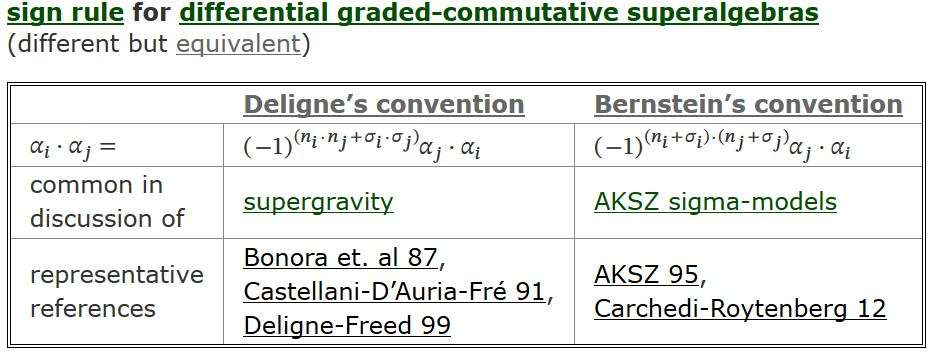$\,$

Special cases of dgc-superalgebras

$\phantom{A}$bi-degree$\phantom{A}$
$\phantom{A}$$(n,\sigma) \in \mathbb{Z} \times \mathbb{F}_2$$\phantom{A}$
$\phantom{A}$$n = 0$$\phantom{A}$$\phantom{A}$$n\;$ arbitrary$\phantom{A}$
$\phantom{A}$$\sigma = even$$\phantom{A}$$\phantom{A}$commutative algebra$\phantom{A}$$\phantom{A}$differential graded-commutative algebra$\phantom{A}$
$\phantom{A}$$\sigma\;$ arbitrary$\phantom{A}$$\phantom{A}$e.g. Grassmann algebra$\phantom{A}$$\phantom{A}$differential graded-commutative superalgebra$\phantom{A}$

$\,$

Example – The Grassmann algebra (Grassmann 1844)

$\phantom{AAA}$$\mathbb{R}\left[ \underset{deg = (0,odd)}{\underbrace{\langle \theta^\alpha \rangle_{\alpha = 1}^q}} \right]$

$\phantom{AAA}$is the free supercommutative superalgebra over $\mathbb{R}$

$\phantom{AAA}$on $q$ odd-graded generators: $\theta^\alpha \theta^\beta = - \theta^\beta \theta^\alpha$

Example – A super Cartesian space $\mathbb{R}^{n\vert q}$

$\phantom{AAA}$is the formal dual space of the tensor product of algebras

$\phantom{AAA}$$C^\infty\left( \mathbb{R}^{n\vert q}\right) \;\coloneqq\; \underset{ {\mathbb{R}\text{-algebra of}} \atop \text{smooth functions} }{ \underbrace{ C^\infty(\mathbb{R}^n) }} \otimes_{\mathbb{R}} \underset{ \text{Grassmann algebra} }{ \underbrace{ \wedge^\bullet_{\mathbb{R}} \mathbb{R}^q }}$

$\,$

$\,$

$\,$

differential forms on super Cartesian space $\mathbb{R}^{n\vert q}$

$\phantom{AAA}$is the differential graded-commutative superalgebra

$\phantom{AAA}$free over $C^\infty\left(\mathbb{R}^{n\vert q}\right)$

$\phantom{AAA}$on

1. $n$ generators $\mathbf{d} x^a$ in bi-degree $(1,even)$

2. $q$ generators $\mathbf{d} \theta^\alpha$ in bi-degree $(1,odd)$

$\,$

hence

$\phantom{AAAAA}$$\Omega^\bullet( \mathbb{R}^{n\vert q} ) \;\coloneqq\; C^\infty(\mathbb{R}^{n\vert q}) \left[ \underset{deg = (1,even)}{\underbrace{ \left\langle \mathbf{d} x^a \right\rangle_{a = 1}^{n} }},\;\;\;\;\; \underset{ deg = (1,odd) }{ \underbrace{ \left\langle \mathbf{d} \theta^\alpha \right\rangle_{\alpha = 1}^q }} \right]$

$\,$

$\,$

$\,$

Bi-Degrees and signs for super differential forms

$\phantom{A}$generator$\phantom{A}$$\phantom{A}$bi-degree$\phantom{A}$
$\phantom{A}$$x^a$$\phantom{A}$$\phantom{A}$(0,even)$\phantom{A}$
$\phantom{A}$$\theta^\alpha$$\phantom{A}$$\phantom{A}$(0,odd)$\phantom{A}$
$\phantom{A}$$\mathbf{d}x^a$$\phantom{A}$$\phantom{A}$(1,even)$\phantom{A}$
$\phantom{A}$$\mathbf{d}\theta^\alpha$$\phantom{A}$$\phantom{A}$(1,odd)$\phantom{A}$

$\,$

$\phantom{A}$sign rule$\phantom{A}$$\phantom{A}$Deligne’s$\phantom{A}$Bernstein’s$\phantom{A}$
$\phantom{A}$$x^{a} \; x^{b} =$$\phantom{A}$$+ x^{b} \; x^{a}$$\phantom{A}$$\phantom{A}$$+ x^{b} \; x^{a}$$\phantom{A}$
$\phantom{A}$$x^a \;\theta^\alpha =$$\phantom{A}$$\phantom{A}$$+ \theta^\alpha \; x^a$$\phantom{A}$$\phantom{A}$$+ \theta^\alpha \; x^a$$\phantom{A}$
$\phantom{A}$$\theta^{\alpha} \; \theta^{\beta} =$$\phantom{A}$$\phantom{A}$$- \theta^{\beta} \; \theta^{\alpha}$$\phantom{A}$$\phantom{A}$$- \theta^{\beta} \; \theta^{\alpha}$$\phantom{A}$
$\phantom{A}$$x^{a} (\mathbf{d}x^{a}) =$$\phantom{A}$$\phantom{A}$$+ (\mathbf{d}x^{b}) x^{a}$$\phantom{A}$$\phantom{A}$$+ (\mathbf{d}x^{b}) x^{a}$$\phantom{A}$
$\phantom{A}$$\theta^\alpha (\mathbf{d}x^a) =$$\phantom{A}$$\phantom{A}$$+ (\mathbf{d}x^a) \theta^\alpha$$\phantom{A}$$\phantom{A}$${\color{blue}{-}} (\mathbf{d}x^a) \theta^\alpha$$\phantom{A}$
$\phantom{A}$$\theta^{\alpha} (\mathbf{d}\theta^{\beta}) =$$\phantom{A}$$\phantom{A}$$- (\mathbf{d}\theta^{\beta}) \theta^{\alpha}$$\phantom{A}$$\phantom{A}$${\color{blue}{+}} (\mathbf{d}\theta^{\beta}) \theta^{\alpha}$$\phantom{A}$
$\phantom{A}$$(\mathbf{d}x^{a}) (\mathbf{d} x^{b}) =$$\phantom{A}$$\phantom{A}$$- (\mathbf{d} x^{b}) (\mathbf{d} x^{a})$$\phantom{A}$$\phantom{A}$$- (\mathbf{d} x^{b}) (\mathbf{d} x^{a})$$\phantom{A}$
$\phantom{A}$$(\mathbf{d}x^a) (\mathbf{d} \theta^{\alpha}) =$$\phantom{A}$$\phantom{A}$$- (\mathbf{d}\theta^{\alpha}) (\mathbf{d} x^a)$$\phantom{A}$$\phantom{A}$${\color{blue}{+}} (\mathbf{d}\theta^{\alpha}) (\mathbf{d} x^a)$$\phantom{A}$
$\phantom{A}$$(\mathbf{d}\theta^{\alpha}) (\mathbf{d} \theta^{\beta}) =$$\phantom{A}$$+ (\mathbf{d}\theta^{\beta}) (\mathbf{d} \theta^{\alpha})$$\phantom{A}$$\phantom{A}$$+ (\mathbf{d}\theta^{\beta}) (\mathbf{d} \theta^{\alpha})$$\phantom{A}$

$\,$

$\,$

pullback of super differential forms

$\phantom{AAA}$Each morphism

$\phantom{AAA}$$\array{ \mathbb{R}^{n_1 \vert q_1} &\overset{\phantom{A}f\phantom{A}}{\longrightarrow}& \mathbb{R}^{n_2 \vert q_2} \\ C^\infty(\mathbb{R}^{n_1\vert q_1}) &\overset{\phantom{A}f^\ast\phantom{A}}{\longleftarrow}& C^\infty(\mathbb{R}^{n_2\vert q_2}) }$

$\phantom{AAA}$has unique dg-extension

$\phantom{AAAAA}$$f^\ast \circ \mathbf{d}_2 = \mathbf{d}_1 \circ f^\ast \phantom{AAA} \array{ \Omega^\bullet(\mathbb{R}^{n_1\vert q_1}) &\overset{\phantom{AA}f^\ast\phantom{AA}}{\longleftarrow}& \Omega^\bullet(\mathbb{R}^{n_2\vert q_2}) \\ {}^{\mathbf{d}_1}\Big\downarrow && \Big\downarrow{}^{\mathbf{d}_2} \\ \Omega^\bullet(\mathbb{R}^{n_1\vert q_1}) &\overset{\phantom{AA}f^\ast\phantom{AA}}{\longleftarrow}& \Omega^\bullet(\mathbb{R}^{n_2\vert q_2}) }$

$\,$

$\phantom{AAA}$This defines another fully faithful functor

$\phantom{AAAAA}$$\phantom{AAA}$$\mathbf{\Omega}^\bullet \;\colon\; SuperCartSp \overset{\phantom{AAAA}}{\hookrightarrow} dgcSuperAlg^{op}$

$\,$

$\,$

$\,$

$\,$

For $\mathfrak{g}$ a super Lie algebra of finite dimension,

$\phantom{AAA}$is the differential graded-commutative superalgebra

$\phantom{AAAAA}$$CE(\mathfrak{g}) \;\coloneqq\; \mathbb{R} \left[ \underset{ (1,\bullet) }{\underbrace{\mathfrak{g}^\ast}} \right]$

$\phantom{AAA}$equipped with the differential $d_{\mathfrak{g}}$

$\phantom{AAA}$which is the linear dual of the super Lie bracket

$\phantom{AAA}$$d_{\mathfrak{g}} \coloneqq [-,-]^\ast \;\colon\; \mathfrak{g}^\ast \to \mathfrak{g}^\ast \wedge \mathfrak{g}^\ast$

$\,$

Equivalently:

$\phantom{AAAAA}$$\phantom{AA}$$CE(\mathfrak{g}) \;\simeq\; \Omega^\bullet_{li}( G )$

$\phantom{AAA}$is the dgc-superalgebra of left-invariant super differential forms

$\phantom{AAA}$on the corresponding simply connected super Lie group.

$\,$

$\,$

$\,$

key Example – super Minkowski spacetime

$\phantom{AAA}$For $d \in \mathbb{N}$

$\phantom{AAA}$and $\mathbf{N}$ a real spin representation of $Spin(d-1,1)$

$\phantom{AAA}$the super-translation supersymmetry super Lie algebra

$\phantom{AAAAA}$$\mathbb{R}^{d-1,1\vert \mathbf{N}}$

$\phantom{AAA}$has Chevalley-Eilenberg algebra generated by

$\phantom{AAAAA}$$\left\{\; \underset{(1,even)}{\underbrace{ \phantom{A}e^a\phantom{A} }}\; \right\}_{a = 0}^{d-1} \phantom{AAA} \left\{\; \underset{(1,odd)}{\underbrace{ \phantom{A}\psi^\alpha \phantom{A} }} \; \right\}_{\alpha = 1}^N$

$\phantom{AAA}$with differential

$\phantom{AAAAA}$\begin{aligned} d \, \psi_\alpha &\;=\; 0 \\ d\, e^a & \;=\; \underset{ \text{spinor-to-vector} \atop \text{pairing} }{ \underbrace{ \overline{\psi} \wedge \Gamma^a \psi }} \end{aligned}

$\,$

$\,$

$\,$

$\,$

$\,$

If we think of super Minkowski spacetime $\mathbb{R}^{d-1,1\vert \mathbf{N}}$

as the super Cartesian space with coordinates

$\phantom{AAAAA}$$\left\{\; \underset{(0,even)}{\underbrace{ \phantom{A}x^a\phantom{A} }}\; \right\}_{a = 0}^{d-1} \phantom{AAA} \left\{\; \underset{(0,odd)}{\underbrace{ \phantom{A}\theta^\alpha \phantom{A} }} \; \right\}_{\alpha = 1}^N$

then these generators correspond to

the super-left invariant

super vielbein

$\phantom{AAAAA}$\begin{aligned} \psi^\alpha & \;=\; d \theta^\alpha \\ e^a & \;=\; \underset{ \text{ordinary} \atop \text{Minkowski vielbein} }{ \underbrace{ \;d x^a\; }} + \underset{\text{correction term} \atop \text{for left super-invariance}}{\underbrace{\;\overline{\theta} \Gamma^a d \theta\;}} \end{aligned}

$\,$

$\,$

$\,$

$\,$

$\,$

$\,$

$\,$

are in natural bijection with

the dual homomorphisms of dgc-superalgebras

between their Chevalley-Eilenberg algebra,

$\phantom{AAAAA}$$\array{ \phantom{CE(}\mathfrak{g}_1\phantom{)} \overset{\phantom{AA}f\phantom{AA}}{\longrightarrow} \phantom{CE(}\mathfrak{g}_2\phantom{)} \\ CE(\mathfrak{g}_1) \underset{\phantom{AA}f^\ast \phantom{AA}}{\longleftarrow} CE(\mathfrak{g}_2) }$

hence we have another fully faithful functor

$\phantom{AAAAA}$$CE \;\colon\; SuperLieAlg \overset{\phantom{AAAA}}{\hookrightarrow} dgcSuperAlg^{op}$

$\,$

$\,$

$\,$

$\,$

$\,$

$\,$

Hence: A super L-∞ algebra of finite type is

1. a $\mathbb{Z}$-graded super vector space $\mathfrak{g}$

degreewise of finite dimension

2. for all $n \geq 1$ a multilinear map

$\phantom{AA}$$[-,\cdots, -] \;\colon\; \wedge^n \mathfrak{g}^\ast \longrightarrow \wedge^1 \mathfrak{g}^\ast$

of degree $(-1,even)$

$\phantom{AAA}$such that the graded derivation

$\phantom{AAAAA}$$d_{\mathfrak{g}} \;\coloneqq\; [-]^\ast + [-,-]^\ast + [-,-,-]^\ast + \cdots \;\;\colon\;\; \wedge^1 \mathfrak{g}^\ast \longrightarrow \wedge^\bullet \mathfrak{g}^\ast$

$\phantom{AAA}$squares to zero: $d_{\mathfrak{g}} d_{\mathfrak{g}} = 0$

This defines a dgc-superalgebra

$\phantom{AAAAA}$$CE(\mathfrak{g}) \coloneqq ( \wedge^\bullet \mathfrak{g}^\ast, d_{\mathfrak{g}} ) \,.$

$\phantom{AAA}$ super L-∞ algebras form the full subcategory

$\phantom{AAAAA}$$Super L_\infty Alg^{fin} \overset{ \phantom{AAA}CE\phantom{AAA} }{\hookrightarrow} dgcSuperAlg^{op}$

$\,$

$\,$

$\,$

Side remarks.

1. We may drop the assumption of degreewise finiteness

by regarding $\vee^\bullet \mathfrak{g}$ as

and $D \coloneqq [-] + [-,-] + [-,-,-] + \cdots$ as a differential

2. The above definition is for non-curved L-∞ algebras

but allows for “curved sh-maps”.

This is important in the discussion of Super topological T-Duality.

(see the talk by D. Fiorenza at the meeting)

3. By allowing algebras of smooth functions in degree 0

we obtain super-L-∞ algebroids (Sati-S.-Stasheff 12, A.1)

formally dual to super dg-manifolds.

$\,$

$\,$

$\,$

convoluted History:

$L_\infty$-algebras were explicitly introduced in Lada-Stasheff 92

but following their discovery by Zwiebach 89, Zwiebach 92 in closed string field theory.

$\,$

The equivalence to Chevalley-Eilenberg dg-(co-)algebras

is due to Lada-Markl 94, see Sati-Schreiber-Stasheff 08, around def. 13.

$\,$

But these dual CE-algebras of super L-∞ algebras of finite type

were introduced already in van Nieuwenhuizen 82, D’Auria-Fré 82

for higher super Cartan geometry of supergravity, there called FDAs.

$\phantom{A}$higher Lie theory$\phantom{A}$$\phantom{A}$supergravity$\phantom{A}$
$\phantom{A}$ super L-∞ algebras $\mathfrak{g}$ $\phantom{A}$$\phantom{A}$FDAs$CE(\mathfrak{g})$ $\phantom{A}$

$\,$

The string theory-community began to pay attention with Hohm-Zwiebach 17

$\,$

$\,$

Higher Lie algebras turn out to be the answer to the question:

What do higher Lie algebra cocycles classify?

$\,$

Recall:

A (p+2)-cocycle on a Lie algebra $(\mathfrak{g},[-,-])$ is

1. multi-linear, skew-symmetric function $\mu_{p+2} \;\colon\; \underset{p+2\;\text{copies}}{\underbrace{\mathfrak{g} \times \cdots \times \mathfrak{g}}} \longrightarrow \mathbb{R}$;

2. cocycle condition: for all $(p+3)$-tuples $(x_0, \cdots, x_{p+2})$ in $\mathfrak{g}$

$\phantom{AA}$$\underset{\sigma\in perm.}{\sum} (-1)^{sgn(\sigma)} \, \mu_{p+2} \left([x_{\sigma_0},x_{\sigma_1}], x_{\sigma_2}, \cdots, x_{\sigma_{p+2}}\right) = 0 \,.$

$\,$

In terms of Chevalley-Eilenberg algebras this means simply

1. $\mu_2 \in CE(\mathfrak{g})$

2. $d \mu_2 = 0$

$\phantom{A}$(this is the classical use of CE-algebras)

$\,$

$\,$

$\,$

A Lie algebra 2-cocycle $\mu_2$

corresponds to a central Lie algebra extension,

$\phantom{AAA}$$\array{ \widehat \mathfrak{g} & \coloneqq \mathfrak{g} \oplus \mathbb{R}, &\phantom{A}& [\, (x_1,c_1),\,(x_2,c_2) \,] \; \coloneqq \; (\, [x_1,x_2], \, \mu_2(x_1,x_2) \,) \\ {}^{\mathllap{p}}\Big\downarrow \\ \mathfrak{g} } \,.$

$\phantom{AAA}$the new Jacobi identity is the cocycle condition on $\mu_2$.

In terms of dual Chevalley-Eilenberg algebras this means simply:

$\phantom{AAA}$$\array{ CE(\widehat{\mathfrak{g}}) & = CE(\mathfrak{g})[ \underset{(1,even)}{\underbrace{\phantom{A} b \phantom{A} }} ], &\phantom{A}& d \,b = \mu_2 \\ {}^{\mathllap{p^\ast}}\Big\uparrow \\ CE(\mathfrak{g}) }$

$\phantom{AAA}$the new nilpotency $d^2 = 0$ is the cocycle condition on $\mu_2$

$\,$

$\,$

$\,$

Non-classical Fact:

A $(p+2)$-cocycle $\mu_{p+2}$ still corresponds to

$\phantom{AAA}$$\array{ \widehat{\mathfrak{g}} & = \left[ \cdots \overset{0}{\to} \underset{ (p,even) }{\underbrace{\mathbb{R}}} \overset{0}{\to} \cdots \overset{0}{\to} \mathfrak{g} \right], &\phantom{A}& [x_1, \cdots, x_{p+2}] = \mu_{p+2}(x_1, \cdots, x_{p+2}) \\ {}^{\mathllap{p}}\Big\downarrow \\ \mathfrak{g} }$

$\,$

In terms of dual Chevalley-Eilenberg algebras this means simply

$\phantom{AAA}$$\array{ CE(\widehat{\mathfrak{g}}) & = CE(\mathfrak{g})[ \underset{(p+1,even)}{\underbrace{ \phantom{A}b\phantom{A} }} ] &\phantom{A}& d \, b = \mu_{p+2} \\ {}^{\mathllap{p^\ast}}\Big\uparrow \\ CE(\mathfrak{g}) }$

$\,$

$\,$

$\,$

$\,$

Example – string Lie 2-algebra

$\phantom{AAA}$Every semisimple Lie algebra $\mathfrak{g}$ carries a Killing form pairing

$\phantom{AAAAA}$$\phantom{AA}$$\langle -,- \rangle \;\colon\; \mathfrak{g} \times \mathfrak{g} \longrightarrow \mathfrak{g}$

$\phantom{AAA}$and the resulting trilinear map

$\phantom{AAA}$$\mu_3 \coloneqq \langle -, [-,-] \rangle$

$\phantom{AAA}$is a 3-cocycle.

$\phantom{AAA}$This classifies a Lie 2-algebra extension of $\mathfrak{g}$,

$\phantom{AAA}$called the string Lie 2-algebra

$\phantom{AAA}$(Baez-Crans 03, Baez-Crans-S.-Stevenson 05).

In string theory/M-theory this controls

$\,$

$\,$

Generally: For $\mathfrak{g}$ a super L-∞ algebra

1. an $L_\infty$ $p+2$-cocycle is $\mu_{p+2} \in CE(\mathfrak{g})$,

2. the higher central extension $\widehat \mathfrak{g}$ it classifies has

$CE(\widehat{\mathfrak{g}}) \;=\; CE(\mathfrak{g})[ \underset{(p+1,even)}\underbrace{\;b\;}] / (\; d \, b = \mu_{p+2}\;)$

Hence from any super L-∞ algebra emanates a “bouquet” of consecutive higher central extension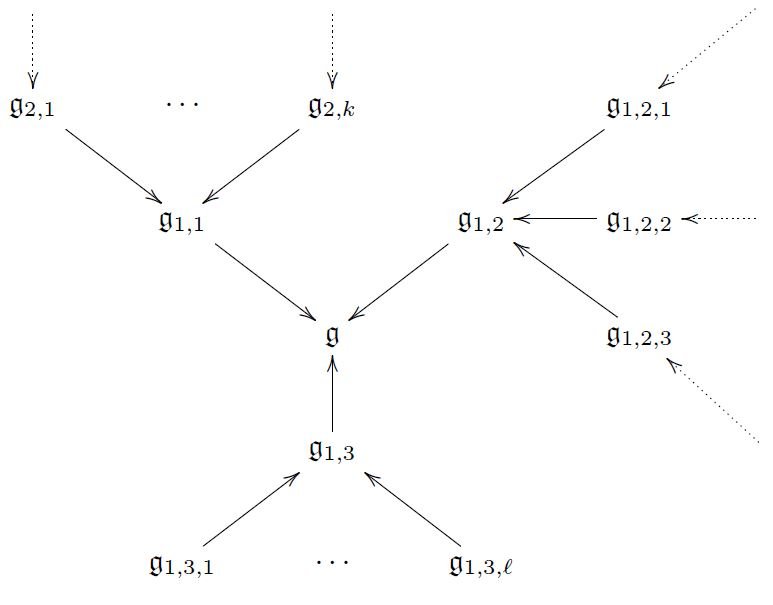$\,$

$\,$

### 3) Homotopy theory

have been founded on set theory,

whose concept of sets is that of “bags of distinguishable points”.

$\,$

But fundamental physics is governed by the gauge principle.

This says that given any two “things”,

such as two field histories $x$ and $y$,

it is in general wrong to ask whether they are equal or not,

$\phantom{AAA}$$x \overset{\phantom{AA}\gamma\phantom{AA}}{\longrightarrow} y$

between them.

In mathematics this is called a homotopy.

$\,$

$\,$

The gauge principle applies to gauge transformations/homotopies themselves,

and thus leads to gauge-of-gauge transformations or homotopies of homotopies

$\phantom{AAA}$and so on to ever higher gauge transformations or higher homotopies:

$\phantom{AAA}$A collection of elements with higher gauge transformations/higher homotopies

is called a higher homotopy type.

$\,$

$\,$

$\,$

Hence the theory of homotopy types

$\phantom{AAA}$homotopy theory

$\phantom{AAA}$is much like set theory,

$\phantom{AAA}$but with the concept of gauge transformation

$\phantom{AAA}$built right into its foundations.

$\,$

$\,$

Homotopy theory is gauged mathematics.

$\,$

$\,$

$\,$

$\,$

$\,$

$\,$

$\,$

A model for homotopy

$\phantom{AAA}$may be given by

$\phantom{AA}$left homotopy$\phantom{AA}$$\phantom{AA}$right homotopy$\phantom{AA}$
$\phantom{AAAA} \array{ X \\ {}^{\mathllap{(id,\delta_0)}}\downarrow & \searrow^{\mathrlap{f}} \\ X \times I &\stackrel{\eta}{\longrightarrow}& Y \\ {}^{\mathllap{(id,\delta_1)}}\uparrow & \nearrow_{\mathrlap{g}} \\ X } \phantom{AA}$$\phantom{AA} \array{ && Y \\ & {}^{\mathllap{f}}\nearrow & \Big\uparrow{}^{\mathrlap{ \Maps(\delta_0,Y) }} \\ X &\overset{\widetilde \eta}{\longrightarrow}& Maps(I,Y) \\ &{}_{\mathllap{g}}\searrow& \Big\downarrow{}^{ \mathrlap{Maps( \delta_1, Y ) } } \\ && Y } \phantom{AAAA}$

$\,$

$\,$

$\,$

$\,$

$\,$

A category $\mathcal{C}$ with a good supply of

1. cylinder objects$X \times I$

2. path space objects$Maps(I,X)$

allowing homotopy theory this way

is called a model category

short for “category of models for homotopy types”.

$\,$

Induced: Its homotopy category $Ho(\mathcal{C})$ whose

1. morphisms are homotopy classes of morphisms in $\mathcal{C}$

$\,$

$\,$

$\,$

$\,$

$\,$

$\,$

Example – topological homotopy theory

$\phantom{AAA}$The category Top of topological spaces

$\phantom{AAA}$becomes a model category, Top${}_{Qu}$

$\phantom{AAA}$via the usual cylinder spaces and path spaces

$\,$

$\,$

$\,$

$\,$

$\,$

$\,$

$\,$

$\,$

$\,$

$\,$

$\,$

Example – dgc-algebraic super homotopy theory

$\phantom{AAA}$The category of real differential graded-commutative superalgebras

$\phantom{AAA}$carries a “projective” model category structure $dgcSuperAlg_{proj}$

$\phantom{AAA}$with path space objects given by tensor product of algebras with $\Omega^\bullet_{poly}\big([0,1]\big)$

$\,$

$\,$

$\,$

$\,$

$\,$

$\,$

$\,$

$\,$

$\,$

$\,$

Example – simplicial homotopy theory

$\phantom{AAA}$An n-simplex is an $n$-dimension generalization of a triangle.

$\phantom{AAA}$A simplicial complex is a space glued from simplices of any dimension.

$\phantom{AAA}$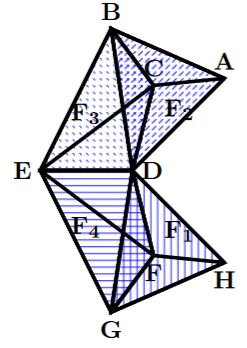$\,$

$\,$

$\,$

Generally, a simplicial set $X$ is

$\phantom{AAA}$for each $n \in \mathbb{N}$ a set $X_n$ of would-be $n$-simplices,

$\phantom{AAA}$and functions between these sets that behave like

1. sending $(n+1)$-simplices to their $n$-dimensional faces,

2. constructing degenerate $(n+1)$-simplices on given $n$-simplices.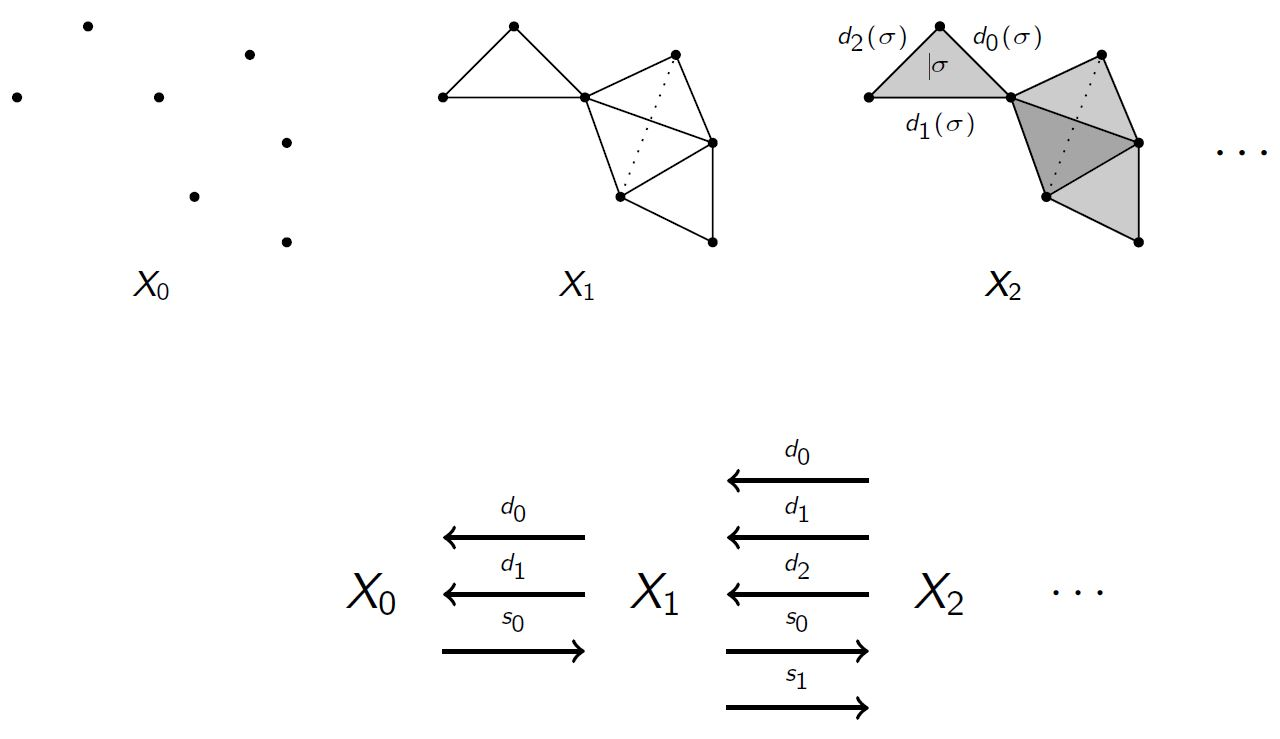$\,$

$\,$

Example – The canonical cylinder object

$\phantom{AAA}$on the 2-simplex look as follows

$\phantom{AAA}$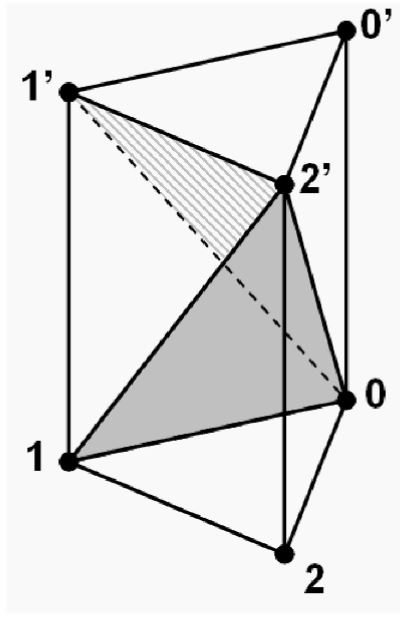graphics grabbed from Friedman 08, p. 33

$\,$

∞-groupoids

A simplicial set is called a Kan complex if

1. its $n$-simplices may be composed

2. each has an inverse under composition, up to $(n+1)$-simplices

These are the good models for simplicial homotopy types.

$\,$

Example – a discrete group $G$, is a Kan complex $B G$ with

1. a single 0-simplex $\phantom{AAAAAAAAAAAa}$$\ast$

2. 1-simplices the group elements $\phantom{AA}$$\ast \overset{\phantom{AA}g\phantom{AA}}{\longrightarrow} \ast$

3. 2-simplices their product $\phantom{AAAAAAa}$$\array{ && \ast\\ & {}^{\mathllap{g_1}}\nearrow && \searrow^{\mathrlap{g_2}} \\ \ast && \underset{g_2 g_1}{\longrightarrow} && \ast }$

4. 3-simplices their associativity $\phantom{AAA}$$\array{ \ast &\overset{ \phantom{AA}g\phantom{AA} }{\longrightarrow}& \ast \\ {}^{\mathllap{f}}\Big\uparrow & \searrow^{\mathrlap{h g}} & \Big\downarrow{}^{\mathrlap{h}} \\ \ast &\underset{ (h g) f }{\longrightarrow}& \ast } \;\;\;\;=\;\;\;\; \array{ \ast &\overset{ \phantom{AA}g\phantom{AA} }{\longrightarrow}& \ast \\ {}^{\mathllap{f}}\Big\uparrow & {}^{\mathllap{ g f }}\nearrow & \Big\downarrow{}^{\mathrlap{h}} \\ \ast &\underset{ h (g f) }{\longrightarrow}& \ast }$

$\,$

$\phantom{AAA}$is called a Quillen adjunction

$\phantom{AAA}$$\mathcal{C} \; \underoverset {\underset{R}{\longrightarrow}} {\overset{L}{\longleftarrow}} {\phantom{{}_{Qu}}\bot_{Qu}} \; \mathcal{D}$

$\phantom{AAA}$if, very roughly (see here)

1. the left adjoint preserves cylinder objects;

2. the right adjoint preserves path space objects.

This induces an adjunction of derived functors on homotopy categories

$\phantom{AAA}$$Ho(\mathcal{C}) \; \underoverset {\underset{\mathbb{R}R}{\longrightarrow}} {\overset{\mathbb{L}L}{\longleftarrow}} {\phantom{AA}\bot\phantom{AA}} \; Ho(\mathcal{D})$

$\,$

This is a Quillen equivalence if the derived adjunction is an equivalence of categories

$\phantom{AAA}$$Ho(\mathcal{C}) \; \underoverset {\underset{\mathbb{R}R}{\longrightarrow}} {\overset{\mathbb{L}L}{\longleftarrow}} {\phantom{AA}\simeq\phantom{AA}} \; Ho(\mathcal{D})$

$\,$

$\,$

$\,$

Example

simplicial and topological homotopy theory are Quillen equivalent

$\phantom{AAAAA}$$Top_{Qu} \; \underoverset {\underset{Sing}{\longrightarrow}} {\overset{{\vert-\vert}}{\longleftarrow}} {\phantom{A}\phantom{{}_{Qu}}\simeq_{Qu}\phantom{A}} \; sSet_{Qu}$

$\,$

$\phantom{AAAAA}$$Ho(Top) \; \underoverset {\underset{\mathbb{R}Sing}{\longrightarrow}} {\overset{\mathbb{L}{\vert-\vert}}{\longleftarrow}} {\phantom{AA}\simeq\phantom{AA}} \; Ho(sSet)$

via forming

• geometric realization${\vert-\vert}$ sending abstract $n$-simplices to topological $n$-simplices $\Delta^n$;

• singular simplicial complexes: $Sing(X)_n \coloneqq Hom_{Top}(\Delta^n,X)$.

Hence both represent

$\,$

$\,$

$\,$

Remarkable richness of homotopy theory:

For a pointed object $\ast \overset{a}{\to} A$ and a morphism $X \overset{c}{\longrightarrow} A$

define the homotopy fiber $\widehat X \overset{hofib(c)}{\longrightarrow} X$ of $c$ over $a$ by:

1. $\hat X$ is in the fiber, up to homotopy: $\phantom{AA}$$\phantom{hofib(c)}$$\array{ \hat X &\overset{\phantom{AA}\phantom{c}\phantom{AA}}{\longrightarrow} & \ast \\ {}^{\mathllap{hofib(c)}}\Big\downarrow &\swArrow& \Big\downarrow\\ X &\underset{\phantom{AA} c \phantom{AA}}{\longrightarrow}& A }$

2. $\hat X$ is universal with this property.

$\array{ \cdots &\longrightarrow& \Omega^2 A &\overset{}{\longrightarrow}& \Omega \widehat X \\ && && {}^{\mathllap{ \Omega hofib(c) }}\Big\downarrow \\ && &&\Omega X &\overset{\phantom{A} \Omega c \phantom{A}}{\longrightarrow}& \Omega A &\overset{ \text{connecting} \atop \text{homomorphism} }{\longrightarrow}& \widehat X & { \text{loop space} \atop \phantom{A} } \\ && && && && {}^{\mathllap{hofib(c)}}\Big\downarrow \\ && && && && X &\underset{\phantom{AA}c\phantom{AA}}{\longrightarrow}& A }$

$\,$

$\,$

$\,$

example:

higher central extensions $\widehat{\mathfrak{g}}$ of super L-∞ algebras $\mathfrak{g}$

are homotopy fibers of the corresponding cocycles $\mu_{p+2}$:

$\,$

$\,$

$\phantom{AAAAAAAAA}$$\array{ \widehat {\mathfrak{g}} \\ {}^{\mathllap{hofib\left(\mu_{p+2}\right)}}\Big\downarrow \\ \mathfrak{g} &\underset{\mu_{p+2}}{\longrightarrow}& B^{p+1} \mathbb{R} } \phantom{AAAAA} \overset{\phantom{AA}CE\phantom{AA}}{\mapsto} \phantom{AAAAA} \array{ CE(\mathfrak{g})[ \underset{(p+1,even)}{\underbrace{\,b\,}} ]/(d \,b = \mu_{p+2}) \\ \Big\uparrow \\ CE(\mathfrak{g}) &\underset{c \mapsto \mu_{p+2}}{\longleftarrow}& \mathbb{R}[ \underset{(p+2, even)}{\underbrace{\;c\;}} ] }$

$\,$

$\,$

$\,$

$\,$

$\,$

$\,$

### 4) Higher super geometry

$\,$

So far we have established two ingredients:

$\,$

1. realization of the Pauli exclusion principle:

$\,$

2. realization of the gauge principle:

notably of geometrically discrete ∞-groupoids (Kan complexes)

$\,$

Now to combine this to

supergeometric ∞-groupoids (“∞-stacks”) $\phantom{AA}$in$\phantom{AA}$ higher supergeometry.

$\,$

$\,$

$\,$

Consider any category of affine spaces as above

$AffineSpaces \;=\; \left\{ \array{ AffineSchemes, \\ CartesianSpaces, \\ SuperCartesianSpaces, \\ \cdots } \right.$

$\,$

We may bootstrap a notion of generalized spaces:

Geometry as seen by classical sigma-models:

$\phantom{AAA}$To determine a generalized target space $\mathbf{X}$

$\phantom{AAA}$…consider for each brane worldvolume

$\phantom{AAAAA}$ $\Sigma \in AffineSpaces$

$\phantom{AAA}$the collection of sigma-model fields

$\phantom{AAAAA}$$\left\{ \; \Sigma \overset{\phantom{AA}\phi\phantom{AA}}{\longrightarrow} \mathbf{X} \; \right\} \phantom{ {A \atop A} \atop {A \atop A} }$

$\,$

$\,$

Minimum consistency conditions.

1. For every $\Sigma \;\in\; AffineSpaces$, there should be a simplicial set

“of sigma-model fields into $\mathbf{X}$ and their gauge-of-gauge equivalences

$\phantom{AAA}$$\array{ \Sigma &\mapsto& \text{"Maps(}\Sigma,\mathbf{X}\text{)"} }$

quotation marks because $\mathbf{X}$ is yet to exist, will be removed in a moment

2. For every morphism $\phi$ in $AffineSpaces$ there should be a function

precomposition of sigma-model fields with $\phi$”:

$\phantom{AAA}$$\array{ \Sigma_1 &\mapsto& \text{"Maps(}\Sigma_1,\mathbf{X}\text{)"} \\ {}^{\mathllap{\phi}}\Big\downarrow && \Big\uparrow{}^{ \mathrlap{ \;\;\text{"precomposition with}\; \phi\text{"} } } \\ \Sigma_2 &\mapsto& \text{"Maps(}\Sigma_2,\mathbf{X}\text{)"} }$

3. Compatibility with composition of morphisms in $AffineSpaces$.

$\,$

$\,$

$\,$

$\,$

In the language of category theory this is simply summarized by:

$\,$

Classical$\,$ sigma-models see generalized spaces $\mathbf{X}$

$\phantom{AAA}$as functors of the form

$\phantom{AAA}$$\mathbf{X} \;\colon\; AffineSpaces^{op} \longrightarrow sSet \,,$

$\phantom{AAA}$also called simplicial presheaves on $AffineSpaces$.

$\,$

Hence the category of generalized spaces

$\phantom{AAA}$should be a full subcategory of the functor category

$\phantom{AAAAA}$$GeneralizedSpaces \;\subset\; Funct\left(AffineSpaces^{op}, sSet\right)$

$\phantom{AAA}$also called the simplical presheaf topos over $AffineSpaces$.

$\,$

This is naturally a model category: the global model structure on simplicial presheaves:

$\phantom{AAA}$$\phantom{AAA}$$Funct(AffineSpaces^{op}, sSet_{Qu})_{glob}$

$\,$

$\,$

$\,$

key bootstrap theorem of functorial geometry says that

$\phantom{AAA}$ the wish becomes true; we may remove the quotation marks:

$\phantom{AAAAA}$$Hom_{GeneralSpaces}( \Sigma, \mathbf{X} ) \;\simeq\; \mathbf{X}(\Sigma) \;=\; \text{"Maps(}\Sigma,\mathbf{X}\text{)"}$

$\phantom{AAA}$known as the Yoneda lemma.

$\,$

Direct consequence is the key consistency result

$\phantom{AAAAA}$$Hom_{AffineSpaces}(\Sigma_1,\Sigma_2) \;\simeq\; Hom_{GeneralizedSpaces}(\Sigma_1,\Sigma_2)$

$\phantom{AAA}$hence a full subcategory embedding

$\phantom{AAAAA}$$AffineSpaces \overset{\phantom{AAAA}}{\hookrightarrow} GeneralizedSpaces$

$\phantom{AAA}$called the Yoneda embedding.

$\,$

$\,$

$\,$

$\,$

Impose one more condition: locality.

$\,$

$\phantom{AAA}$If $\Sigma \in AffineSpaces$ is covered $\phantom{AA}$ $\array{ U_{i_1} && U_{i_2} && U_{i_3} & \cdots \\ & \searrow & \Big\downarrow & \swarrow \\ && \Sigma }$

$\phantom{AAA}$ by patches $U_i \in AffineSpaces$

$\phantom{AAA}$then a decent generalized space $\mathbf{X}$ should satisfy:

$\phantom{AAAAA}$$\Big\{ \Sigma \overset{\phantom{AA}\phi\phantom{AA}}{\longrightarrow} \mathbf{X} \Big\} \;\underset{\text{weak} \atop \text{homotopy equivalence}}{\simeq}\; \left\{ \array{ \text{tuples of maps}\; U_i \to \mathbf{X} \\ \text{that agree on intersections, up to gauge transformations} \\ \text{which are compatible, up to gauge-of-gauge-transformations } \\ \text{ and so on } } \right\}$

$\,$

If $\mathbf{X}$ satisfies this locality condition

it is called an ∞-stack or (∞,1)-sheaf over $AffineSpaces$.

$\,$

$\,$

$\,$

$\phantom{AAA}$and a Quillen adjunction (“Bousfield localization”)

$\phantom{AAA}$$\underset{ \text{local model structure} }{ \underbrace{ Funct(AffineSpaces,sSet)_{loc} }} \;\; \underoverset {\underset{id}{\longrightarrow}} {\overset{id}{\longleftarrow}} {\phantom{AA}\phantom{{}_{Qu}}\bot_{Qu}\phantom{AA}} \;\; Funct(AffineSpaces,sSet)_{proj}$

$\phantom{AAA}$whose derived adjunction on homotopy categories

$\phantom{AAA}$is the full subcategory inclusion on the ∞-stacks

$\phantom{AAAAA}$$\overset{ Ho\left(\, Sh_\infty(AffineSpaces) \, \right) \;\coloneqq\; }{ \overbrace{ Ho\left(Funct(AffineSpaces,sSet)\right)_{loc} }} \; \; \underoverset {\underset{}{\hookrightarrow}} {\overset{\text{∞-stackification}}{\longleftarrow}} {\bot} \;\; Ho\left(Funct(AffineSpaces,sSet)\right)$

$\,$

This homotopy theory is called the

$\phantom{AAA}$∞-stack ∞-topos over $AffineSpaces$

or

$\phantom{AAA}$(∞,1)-sheaf ∞-topos over $AffineSpaces$

$\,$

$\,$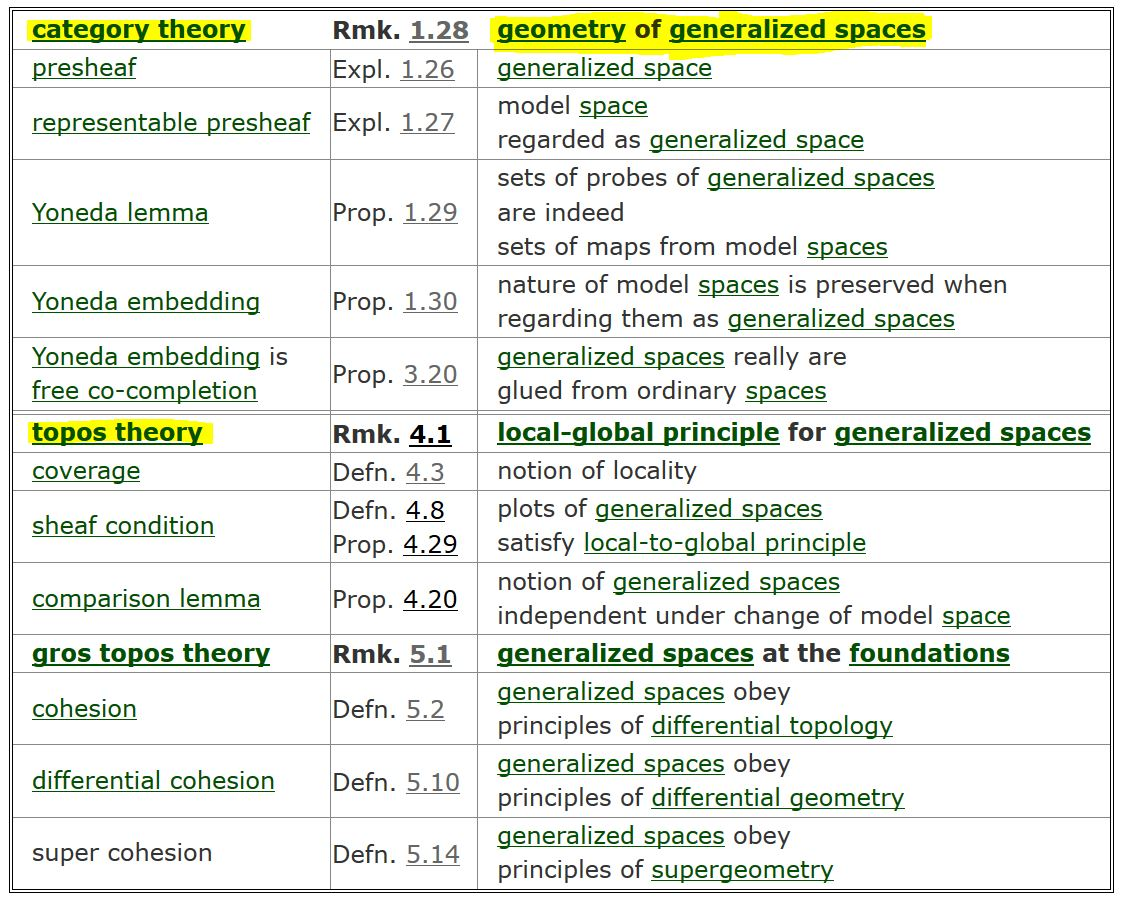$\,$

$\,$

Now to get higher supergeometry

$\phantom{AAA}$ specify to $AffineSpaces \;\coloneqq\;$ SuperCartesianSpaces

$\,$

$\phantom{AAA}$$Ho\left( \, SuperSpaces \, \right) \;\coloneqq\; Ho\big( \, Sh_\infty( \, SuperCartesianSpaces \, ) \, \big)$

$\,$

$\,$

$\,$

$\,$

$\,$

$\,$

this subsumes a rich amount of particular geometries

discussed in the literature:

$\,$

$\,$$\,$

$\,$

### 5) Higher super Lie theory

$\,$

In particular we have a variety of notions of geometric symmetry groups

$\phantom{AAA}$Lie groups $\overset{\phantom{AAAA}}{\hookrightarrow}$ smooth ∞-groups

$\phantom{AAA}$Lie groupoids $\overset{\phantom{AAAA}}{\hookrightarrow}$ smooth ∞-groupoids

$\phantom{AAA}$super Lie groups $\overset{\phantom{AAAA}}{\hookrightarrow}$ super smooth ∞-groups

$\,$

these should be related to

the super L-∞ algebroids discussed above

$\,$

$\,$

$\,$

$\,$

idea (due to Ševera 01,

$\phantom{AAA}$see also Pavol Ševera‘s talk at the meeting):

$\,$

generalize the path method of Lie integration:

$\phantom{AAA}$For $\mathfrak{g}$ a Lie algebra

$\phantom{AAA}$and $G$ its simply connected Lie group

$\phantom{AAA}$observe that parallel transport

$\phantom{AAA}$(after a choice of base point)

$\phantom{AAA}$provides a bijection

$\phantom{AAAAA}$$\underset{ \Omega^1_{flat}\left( \mathbb{R}^n, \mathfrak{g} \right) }{ \underbrace{ Hom_{dgcAlgebras} \left( CE(\mathfrak{g})\;,\; \Omega^\bullet(\mathbb{R}^{n}) \right) }} \underoverset {\simeq} {\phantom{AA}{\text{parallel} \atop \text{transport}}\phantom{AA}} {\longrightarrow} Hom_{SmoothManifolds} \left( \mathbb{R}^{n} \,,\, G \right)$

$\,$

Hence we recover $G$ via smooth homotopy theory as

$\phantom{AAAAA}$$G \;\;\simeq\;\; Maps\big( [0,1]\,, \, \mathbf{\Omega}_{flat}(-,\mathfrak{g}) \big)\,/\,homotopy$

$\,$

$\,$

$\,$

to generalize this

$\phantom{AAA}$recall the functor of super differential forms

$\phantom{AAAAA}$$\mathbf{\Omega}^\bullet \;\colon\; SuperCartesianSpaces \longrightarrow dgcSuperAlgebras^{op}$

$\,$

Consider its enhancement to smooth differential forms on simplices:

$\phantom{AAAA}$$\mathbf{\Omega}^\bullet_{vert, si} \;\colon\; (\mathbb{R}^{n\vert q}, k) \;\mapsto\; \mathbf{\Omega}^\bullet_{vert,si}\left( \mathbb{R}^{n\vert q} \times \Delta^k_{mfd} \right)$

$\phantom{A}$where

1. $\Delta^k$ is the standard k-simplex as a smooth manifold with boundaries and corners;

2. “vert” = vertical differential forms: all legs along the k-simplex;

3. “si” = forms with sitting instants: constant towards boundary of k-simplex

$\,$

$\,$

$\,$

$\,$

higher super Lie integration via higher super path method

for $\mathfrak{g}$ a super L-∞ algebra

with Chevalley-Eilenberg algebra $CE(\mathfrak{g}) \in dgcSuperAlgebras$

Let

$\phantom{AAA}$$Spec(CE(\mathfrak{g})) \;\in\; Ho\left( \,Sh_\infty(\,SuperCartSp\,)\, \right) \;=\; Ho\left( \,SuperSpaces\, \right)$

be given by the simplicial presheaf

$\phantom{AAA}$$Spec(CE(\mathfrak{g}))_k \;\;\colon\;\; \mathbb{R}^{n\vert q} \;\;\mapsto\;\; Hom_{dgcSuperalgebras} \left( CE(\mathfrak{g})\,,\, \Omega^\bullet_{vert,si}\left( \mathbb{R}^{n\vert q} \times \Delta^k_{mfd} \right) \right)$

$\,$

$\,$

example:

For $\mathfrak{g}$ a Lie algebra with simply connected Lie group $G$, we recover

$\tau_1 Spec(CE(\mathfrak{g})) \;\simeq\; \mathbf{B}G \;\in\; Ho\left( \, SuperSpaces \, \right)$

$\,$

$\,$

$\,$

$\,$

$\,$

Theorem (Braunack-Mayer 18)

higher super Lie integration is part of a Quillen adjunction

$\phantom{AAA}$$dgcSuperAlgebras^{op}_{\geq 0, proj} \; \underoverset {\underset{ Spec }{\longrightarrow}} {\overset{ \mathcal{O} }{\longleftarrow}} {\phantom{A}\phantom{{}_{Qu}}\bot_{Qu}\phantom{A}} \; [SuperCartSp^{op},sSet_{Qu}]_{loc}$

$\,$

hence induces a derived adjunction on homotopy categories:

$\phantom{AAA}$$Ho\left(\, dgcSuperAlgebras^{op}_{\geq 0} \, \right) \; \underoverset {\underset{ \mathbb{R} Spec }{\longrightarrow}} {\overset{ \mathbb{L} \mathcal{O} }{\longleftarrow}} {\phantom{A}\phantom{{}_{Qu}}\bot\phantom{_{Qu}}\phantom{A}} \; Ho\left( \, SuperSpaces \, \right)$

$\,$

$\,$

$\,$

$\,$

$\,$

Theorem (Braunack-Mayer 18)

higher super Lie integration restricts to the Sullivan de Rham adjunction

$\underset{ \color{blue} \text{Sullivan de Rham adjunction} }{ \underbrace{ \underset{\phantom{A} \atop \phantom{A}}{ dgcSuperAlg^{op}_{\mathbb{R}, \geq 0, proj} \; \underset{ \color{blue} {\text{higher super} \atop \text{Lie integration} } }{ \underbrace{ \underset{ \phantom{A} \atop \phantom{A} }{ \underoverset {\underset{ Spec }{\longrightarrow}} {\overset{ \mathcal{O} }{\longleftarrow}} {\phantom{A}\phantom{{}_{Qu}}\bot_{Qu}\phantom{A}} }}} \; Funct\left( SuperCartSp^{op},sSet_{Qu}\right)_{loc} \; \overset{ \color{blue}{ \text{include } \atop { \text{geometrically discrete} \atop \text{∞-groupoids} }} }{ \overbrace{ \overset{ \phantom{A} \atop \phantom{A} }{ \underoverset {\underset{\Gamma}{\longrightarrow}} {\overset{const}{\longleftarrow}} {\phantom{A}\phantom{{}_{Qu}}\bot_{Qu}\phantom{A}} }}} \; sSet_{Qu} }} } \; \overset{ \color{blue}{ \text{regard ∞-groupoids} \atop \text{as topological spaces} } }{ \overbrace{ \overset{ \phantom{A} \atop \phantom{A} }{ \underoverset {\underset{Sing}{\longleftarrow}} {\overset{{\vert- \vert}}{\longrightarrow}} {\phantom{A}\phantom{{}_{Qu}}\simeq_{Qu}\phantom{A}} }}} \; Top_{Qu}$

$\,$

classical fact of rational homotopy theory:

$\phantom{AAA}$the derived adjunction restricts to an equivalence of categories

$\phantom{AAAAA}$$\underset{ \color{blue}{ \text{bosonic, connective, finite type algebras} } }{ \underbrace{ Ho\left(\, dgcSuperAlg^{op} \, \right)_{bos, conn, fin} }} \; \; \underoverset {\underset{ \mathbb{R} ( \Gamma \circ Spec ) }{\longrightarrow}} {\overset{ \mathbb{L}( \mathcal{O} \circ const ) }{\longleftarrow}} { \phantom{AA}\simeq\phantom{AA} } \; \; \underset{ \color{blue}{ \text{rational, connected, nilpotent, finite type spaces} } }{ \underbrace{ Ho\left( \, TopologicalSpaces \, \right)_{\mathbb{Q},conn, nil, fin} }}$

(for more on this see D. Fiorenza‘s and V. Braunack-Mayer’s talks at the meeting)

$\,$

$\,$

This implies that

$\,$

higher super Lie integration preserves homotopy fiber sequences

hence in particular higher central extensions

$\,$

$\phantom{AAAA}$$\array{ Super L_\infty Algebras &\overset{ Spec\big(CE(-)\big) }{\longrightarrow}& SuperSpaces \\ \phantom{A} \\ \array{ \widehat{\mathfrak{g}} \\ {}^{\mathllap{hofib\left(\mu_{p+2}\right)}}\Big\downarrow \\ \\ \mathfrak{g} &\underset{ \phantom{A}\mu_{p+2}\phantom{A} }{\longrightarrow}& B^{p+1} \mathbb{R} } & \phantom{AAAAAAAA} \overset{ \phantom{AAAA} }{\mapsto} \phantom{AAAAAAAA} & \array{ \mathbf{B} \widehat{G} \\ {}^{\mathllap{hofib\left(\mathbf{c}_{p+2}\right)}}\Big\downarrow \\ \mathbf{B}G &\underset{ \mathbf{c}_{p+2} }{\longrightarrow}& \mathbf{B}^{p+2} \mathbb{R} } }$

$\,$

$\,$

$\,$

$\,$

$\,$

### 6) Emergence of M-theory

$\,$

homotopy theory reveals rich inner structure of god-given objects

Already the homotopy integers – known as the sphere spectrum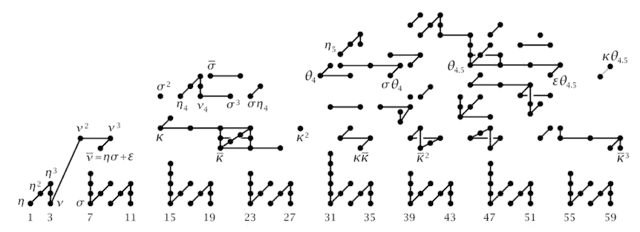(the 2-primary components of the first few stable homotopy groups of spheres)

$\,$

$\,$

$\,$

The microscope of homotopy theory is the Postnikov-Whitehead tower

$\array{ & \mathbf{\text{Whitehead} \atop \text{tower}} \\ &\vdots \\ & B Fivebrane &\to& \cdots &\to& * \\ & \Big\downarrow && \swArrow && \Big\downarrow \\ \mathbf{\text{second fractional} \atop \text{Pontryagin class}} & B String &\to& \cdots &\stackrel{\tfrac{1}{6}p_2}{\to}& B^8 \mathbb{Z} &\overset{\phantom{AAA}}{\longrightarrow}& * \\ & \Big\downarrow && && \Big\downarrow &\swArrow& \Big\downarrow \\ \mathbf{\text{first fractional} \atop \text{Pontryagin class}} & B Spin && \swArrow && &\stackrel{\phantom{A}\tfrac{1}{2}p_1\phantom{A}}{\to}& B^4 \mathbb{Z} &\to & * \\ & \Big\downarrow && && \Big\downarrow &\swArrow& \Big\downarrow &\swArrow& \Big\downarrow \\ \mathbf{\text{second} \atop \text{Stiefel-Whitney class}} & B S O &\overset{}{\to}& \cdots &\overset{}{\to}& &\overset{\phantom{AAA}}{\longrightarrow}& & \overset{\phantom{AA}w_2\phantom{AA}}{\longrightarrow} & \mathbf{B}^2 \mathbb{Z}_2 &\overset{\phantom{AAA}}{\longrightarrow}& * \\ & \Big\downarrow && \swArrow && \Big\downarrow &\swArrow& \Big\downarrow &\swArrow& \Big\downarrow &\swArrow& \Big\downarrow \\ \mathbf{\text{first} \atop \text{Stiefel-Whitney class}} & B O &\to& \cdots &\to& \tau_{\leq 8 } B O &\underset{\phantom{AAAA}}{\longrightarrow}& \tau_{\leq 4 } B O &\underset{\phantom{AAAA}}{\longrightarrow}& \tau_{\leq 2 } B O &\overset{\phantom{A}w_1\phantom{A}}{\longrightarrow}& \tau_{\leq 1 } B O \simeq B \mathbb{Z}_2 & \mathbf{\text{Postnikov} \atop \text{tower}} }$

where each rectangle is a homotopy fiber square.

$\,$

Under higher Lie integration this corresponds to

$\,$

$\array{ \mathfrak{ninebrane} \\ {}^{\mathllap{ hofib\left( \mu_{11} \right) }}\Big\downarrow \\ \mathfrak{fivebrane} &\overset{ \mu_{11} =\langle -,[-,-], [-,-], [-,-], [-,-], [-,-]\rangle }{\longrightarrow}& B^{10} \mathbb{R} \\ {}^{\mathllap{ hofib\left( \mu_7 \right) }}\Big\downarrow \\ \mathfrak{string} &\overset{ \phantom{[-,-]} \mu_7 = \langle -,[-,-], [-,-], [-,-]\rangle \phantom{[-,-]} }{\longrightarrow}& B^6 \mathbb{R} \\ {}^{\mathllap{hofib\left(\mu_3\right)}}\Big\downarrow \\ \mathfrak{so} &\overset{ \phantom{[-,-]} \phantom{[-,-]} \mu_3 = \langle -,[-,-]\rangle \phantom{[-,-]} \phantom{[-,-]} }{\longrightarrow}& B^2 \mathbb{R} }$

$\,$

As the terminology indicates (string Lie 2-algebra, fivebrane Lie 6-algebra,…),

pure homotopy theory is beginning to see string theory-structure…

$\,$

$\,$

To get to the bottom of this

apply this microscope to the superpoint $\mathbb{R}^{0\vert 1}$

$\phantom{AAA}$regarded as a super Lie algebra, hence as a higher superspace

$\phantom{AAA}$$\array{ super L_\infty Algebras &\overset{ \phantom{AA} CE \phantom{AA} }{\longrightarrow}& dgcSuperAlgebras^{op} &\overset{ \phantom{A} Spec \phantom{A} }{\longrightarrow}& Ho\left( \,SuperSpaces\, \right) \\ \mathbb{R}^{0\vert 1} &\mapsto& \mathbb{R} [ \underset{(1,odd) }{\underbrace{\,d \theta\,}} ] &\mapsto& \mathbf{B} \mathbb{R}^{0\vert 1} }$

$\,$

Consider iteratively the

$\phantom{AAA}$invariant universal higher central extensions,

$\phantom{AAA}$at each stage invariant with respect to automorphisms modulo R-symmetries

$\,$

Then the following brane bouquet is revealed…

$\,$

$\phantom{AAA}$see the talks by H. Sati, V. Braunack-Mayer, D. Fiorenza, J. Huerta (here) at the meeting):

$\,$

$\,$

$\phantom{AAA}$$\,$

$\,$

Hence in super homotopy theory

$\phantom{AAA}$core structure of M-theory emerges

$\phantom{AAA}$out of the superpoint.

$\,$

Does the superpoint itself emerge by itself?

$\,$

$\,$

To see this, we invoke now a yet stronger microscope,

$\phantom{AAA}$that of modal homotopy theory

$\,$

$\,$

$\phantom{AAA}$a fully faithful functor with a left adjoint

$\phantom{AAA}$$\mathbf{H}_{\bigcirc} \; \underoverset {\underset{\iota}{\hookrightarrow}} {\overset{ L }{\longleftarrow}} {\phantom{AA}\bot\phantom{AA}} \; \mathbf{H}$

A modal operator is the resulting endofunctor

$\phantom{AAA}$$\bigcirc \;\coloneqq\; \iota \circ L \;\colon\; \mathbf{H} \to \mathbf{H}$

$\phantom{AAA}$which projects onto $\mathbf{H}_{\bigcirc}$.

$\phantom{AAA}$is a fully faithful functor with a right adjoint

$\phantom{AAA}$$\mathbf{H}_{\Box} \; \underoverset {\underset{ R }{\longleftarrow}} {\overset{\iota}{\hookrightarrow}} {\phantom{AA}\bot\phantom{AA}} \; \mathbf{H}$

A comodal operator is the resulting endofunctor

$\phantom{AAA}$$\Box \;\coloneqq\; \iota \circ R \;\colon\; \mathbf{H} \to \mathbf{H}$

$\phantom{AAA}$which projects onto $\mathbf{H}_{\Box}$.

$\,$

$\,$

similarly in homotopy theory:

$\phantom{AAA}$is Quillen adjunction whose derived adjunction is a reflective subcategory

$\phantom{AAA}$$\array{ \mathcal{C} \underoverset {\underset{R}{\longrightarrow}} {\overset{L}{\longleftarrow}} {\phantom{AA}\phantom{{}_{Qu}}\bot_{Qu}\phantom{AA}} \mathcal{D} \\ \\ Ho(\mathcal{C}) \underoverset {\underset{\mathbb{R}R}{\hookrightarrow}} {\overset{\mathbb{L}L}{\longleftarrow}} {\phantom{AAA}\bot\phantom{AAA}} Ho(\mathcal{D}) }$

$\phantom{AAA}$etc.

$\,$

example: the homotopy localization at an object $\mathbb{A}$,

$\phantom{AAA}$is the reflective subcategory of coefficients

$\phantom{AAA}$ representing $\mathbb{A}$-homotopy invariant cohomology

denote the corresponding modal operator by $\bigcirc\!\!\!\!\!\!\!\!\mathbb{A}$

$\,$

$\,$

$\,$

$\phantom{AAA}$is an adjunction (duality) between

$\phantom{AAA}$a modal operator and a comodal operator

$\phantom{AAA}$$\bigcirc \;\dashv\; \Box$

$\phantom{AAA}$or

$\phantom{AAA}$$\Box \;\dashv\; \bigcirc$

this expresses two dual and opposite subcategories

$\,$

for example

$\phantom{AAA}$$Even \dashv Odd \;\colon\; \mathbb{Z}_{\leq} \longrightarrow \mathbb{Z}_{\leq}$

$\phantom{AAA}$with

$\phantom{AAA}$$Even(n) \;\coloneqq\; 2 \lfloor n/2 \rfloor \phantom{AAA} Odd(n) \;\coloneqq\; 2 \lfloor n/2\rfloor + 1$

$\phantom{AA}$is an adjoint modality,

expressing the dual opposition between even and odd numbers.

$\,$

$\,$

preorder on modal operators

$\phantom{AAA}$by inclusion of the subcategories which they project onto

$\phantom{AAA}$$\bigcirc_1 \lt \bigcirc_2 \phantom{AA}if\phantom{AA} \mathbf{H}_{\bigcirc_1} \subset \mathbf{H}_{\bigcirc_2}$

$\,$

$\phantom{A}$ bottom adjoint modality $\phantom{A}$$\phantom{A}$top adjoint modality$\phantom{A}$
$\phantom{A}$$\emptyset \;\dashv \ast\;$$\phantom{A}$$\phantom{A}$$id \dashv id$$\phantom{A}$

$\,$

everything happens in between:

$\phantom{AAA}$$\array{ id &\;\dashv\;& id \\ \vee && \vee \\ \vdots && \vdots \\ \vee && \vee \\ \emptyset &\;\dashv\;& \ast }$

$\,$

$\,$

$\,$

Given a progression of adjoint modalities

$\array{ \Box_2 &\dashv& \bigcirc_2 \\ \vee && \vee \\ \Box_1 &\dashv& \bigcirc_1 }$

we say it provides

sublation of $\Box_1 \dashv \bigcirc_1$

1. $\Box_1 \lt \bigcirc_2$ $\phantom{AAA}$ denoted $\phantom{AAA}$ $\array{ \Box_2 &\dashv& \bigcirc_2 \\ \vee &/& \vee \\ \Box_1 &\dashv& \bigcirc_1 }$

$\phantom{A}$

or

2. $\bigcirc_1 \lt \Box_2$ $\phantom{AAA}$ denoted $\phantom{AAA}$ $\array{ \Box_2 &\dashv& \bigcirc_2 \\ \vee &\backslash& \vee \\ \Box_1 &\dashv& \bigcirc_1 }$

$\,$

$\,$

$\,$

$\,$

hence a duality of dualities

is

$\,$

$\phantom{AAA}$$\array{ \lozenge &\dashv& \Box \\ \bot && \bot \\ \Box & \dashv& \bigcirc }$

$\,$

$\phantom{AAA}$$\lozenge \dashv \Box \dashv \bigcirc$

$\,$

$\,$

$\,$

$\,$

$\,$

Proposition

We have a progression of adjoint modalities on $Ho\big($SuperSpaces$\big)$ as follows:

$\,$

$\phantom{AA}$$\array{ id &\dashv& id \\ \vee &\vert& \vee \\ \rightrightarrows &\dashv& \rightsquigarrow &\dashv& {\bigcirc\!\!\!\!\!\!\!\!\mathbb{R}^{0\vert 1}} \\ && \vee &\backslash& \vee \\ && \Re &\dashv& \Im &\dashv& \& \\ && && \vee &\vert& \vee \\ && && ʃ &\dashv& \flat &\dashv& \sharp \\ && && && \vee &/& \vee \\ && && && \emptyset &\dashv& \ast }$

In this way

the superpoint $\mathbb{R}^{0\vert 1}$

emerges from nothing $\emptyset$

by a progression of dual oppositions, their dualities and their sublation.

$\,$

$\,$

$\,$

$\,$

$\,$

For details see the lecture notes

$\,$

$\,$

$\,$

$\,$

$\,$

$\,$

$\,$

$\,$

$\,$

$\,$

$\,$

$\,$

Last revised on July 6, 2020 at 05:04:38. See the history of this page for a list of all contributions to it.